A Note on the Pricing of American Capped Power Put Option | OMICS International
Journal of Business & Financial Affairs

# A Note on the Pricing of American Capped Power Put Option

Yoshitaka Sakagami*

Faculty of Management, Otemon Gakuin University, Osaka Prefecture 567-0008, Japan

*Corresponding Author:
Yoshitaka Sakagami
Faculty of Management
Otemon Gakuin University
Osaka Prefecture 567-0008
Japan
Tel:+81-72-641-9520
E-mail: [email protected]

Received March 17, 2015; Accepted April 28, 2015; Published June 15, 2015

Citation: Sakagami Y (2015) DA Note on the Pricing of American Capped Power Put Option. J Bus Fin Aff 4:139. doi:10.4172/2167-0234.1000139

Copyright: © 2015 Sakagami Y. This is an open-access article distributed under the terms of the Creative Commons Attribution License, which permits unrestricted use, distribution, and reproduction in any medium, provided the original author and source are credited.

Visit for more related articles at Journal of Business & Financial Affairs

#### Abstract

We give an explicit solution to the perpetual American capped power put option pricing problem in the BlackScholes-Merton Model. The approach is mainly based on free-boundary formulation and verification. For completeness we also give an explicit solution to the perpetual American standard power (_ 1) option pricing problem.

#### Keywords

The perpetual American capped power put option; Geo-etric Brownian motion; Free-boundary

#### Introduction

A standard American power put option is a financial contract that allows the holder to sell an asset for a prescribed amount at any time. The price of this asset is raised to some power. The case of power being one corresponds to the usual American put option . For the European power put option, the value of this option is given [2-4]. For the perpetual American power (≥ 1) put option, for completeness, we give the value of this option and the optimal stopping time. A capped power option is a power option whose maximum payoff is set to a prescribed level. For the European capped power put option, the value of this option is given [2-5]. For the perpetual American capped power put option, we give the value of this option and the optimal stopping time. Throughout this note, the approach is mainly based by freeboundary formulation and verification. Only one exception is Theorem 2.2. This note is organized as follows. In Section 2, we explicitly solve the perpetual American power put option pricing problem. In Section 3, we explicitly solve the perpetual American capped power put option pricing problem [6-8].

#### The Perpetual American Power Put Option

The arbitrage-free price of the perpetual American power put option is given by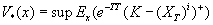(1)

where K is the strike price, T is a stopping time, i is a positive constant greater than or equal 1, and x>0 is the initial value of the stock price process X=(Xt)t ≥ 0. In equation 1, the supremum is taken over all stopping times T of the process X started at x. The stock price process X=(Xt)t ≥ 0 is assumed to be a geometric Brownian motion. That is,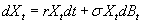(2)

Where ,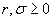.The infinitesimal generator of X is given by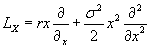(3)

As in the case of standard perpetual American put option, we suppose that there exists a point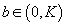such that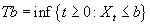(4)

is optimal in equation 1 Then we solve the following free-boundary problem for unknown V and b. Here 0 < bi < K.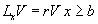(5)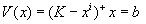(6)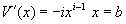(7)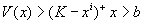(8)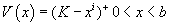(9)

The steps to solve this free-boundary problem is same as in the case of the standard American put option (i=1)  so we only outline the steps. Since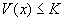, the equation 5 implies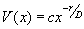(10)

Where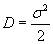and c is an undetermined constant. Using equation 10, we solve two equations 6 and 7 to give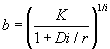(11)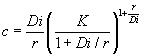(12)

Thus V (x) is written as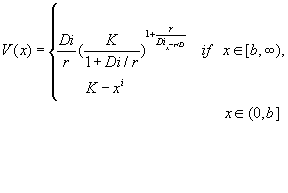(13)

Now we have the following theorem.

Theorem 1.1: V (x) coincides with V*(x), and the optimal stopping time is given by Tb

The steps to prove this theorem is same as in the case of the standard American put option (i = 1) , so we omit.

It should be noted that for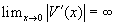,so we assume that i ≥ 1 in this section.

#### The Perpetual American Capped Power Put Option

The arbitrage-free price of the perpetual American capped power put option is given by^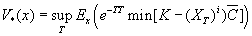(14)

Where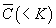and i are positive constants. First we suppose there exists a point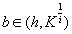such that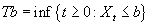(15)

is optimal in (14). Here h satisfies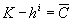. Then we solve the following free-boundary problem for unknown V and b.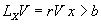(16)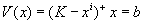(17)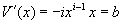(18)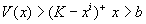(19)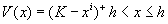(20)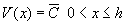(21)

It is clear that for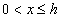the arbitrage-free price from equation14 is given by equation 21. Thus,is the stopping region.

Since we suppose that, b is same as in the case of the American power put option. Thus the free-boundary b is given by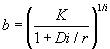(22)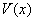is written as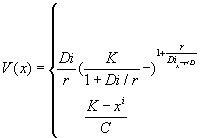if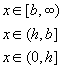(23)

Note that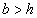is equivalent to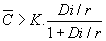Theorem 2.1: Suppose thatthencoincides with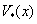and the optimal stopping time is given by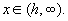Proof: From our earlier consideration we can suppose thatSince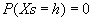and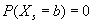, the change-ofvariable formula (see Remark 2.3 in ) with the smooth-fit condition (18) gives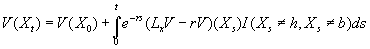(24)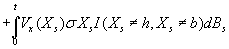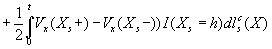S When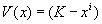, we see that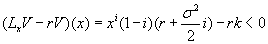for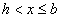. For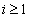,it clearly holds. For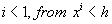it is easily seen to hold. Thus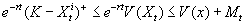Where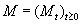is defined by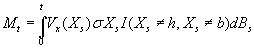is a continuous martingale (because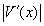is bounded for all x > 0. Thus it is easily verified by standard means (using the localization of M and Fatou’s lemma) that we get that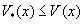For all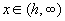Next we set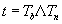in equation 24. Here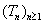is a localization sequence of bounded stopping times for M. For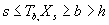.Hence the fourth term in the right-hand side of this equation is zero. Moreover using equation16, we find the second term in the right-hand side is also zero. Finally using the optional sampling theorem we get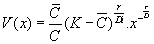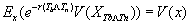(25)

Letting n go to infinity and using the dominated convergence and, we get that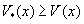for all. The proof is completed.

Theorem 2.2: Suppose that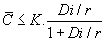then V (x) is written asif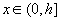(26)

x∈(h,∞)

The optimal stopping time is given by Th .

Proof: We set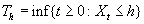. Then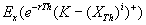(27)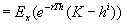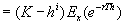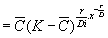(28)

Where the final equality follows by the formula for the expected first hitting time for a geometric Brownian motion

Now we show for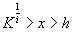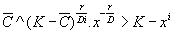(29)

We set f (x) to be equal to the left-hand side minus the right-hand side in equation 29. Clearly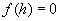To show that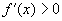, it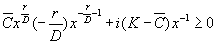(30)

Because x > h . Since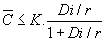(equation30) holds. Since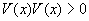equation 29 and 30 imply that (h,∞) is a continuous region. On the other hand, (0,h] is a stopping region. Thus h T is the optimal stopping time and V (x) is given by equation 26. The proof is completed.

#### References

Select your language of interest to view the total content in your interested language

### Article Usage

• Total views: 12119
• [From(publication date):
June-2015 - Feb 19, 2020]
• Breakdown by view type
• HTML page views : 8306Can't read the image? click here to refresh